# Geometric progression

Fill 4 numbers between 4 and -12500 to form geometric progression.

a1:  -20
a2:  100
a3:  -500
a4:  2500

### Step-by-step explanation:

${a}_{2}=4\cdot \left(-5\right)\cdot \left(-5\right)=100$
${a}_{3}=4\cdot \left(-5\right)\cdot \left(-5\right)\cdot \left(-5\right)=-500$
${a}_{4}=4\cdot \left(-5\right)\cdot \left(-5\right)\cdot \left(-5\right)\cdot \left(-5\right)=2500$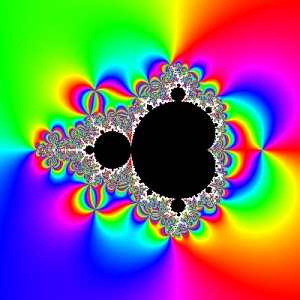Did you find an error or inaccuracy? Feel free to write us. Thank you!## Related math problems and questions:

• InsertInsert five numbers between 8 and 27 such numbers that, with two given ones, they form the first seven members of the geometric sequence.
• Geometric progression 48,4√2,4,2√2
• Insert into GPBetween numbers 5 and 640, insert as many numbers to form geometric progression so the sum of the numbers you entered will be 630. How many numbers must you insert?
• Gp - 80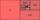One of the first four members of a geometric progression is 80. Find its if we know that the fourth member is nine times greater than the second.
• Geometric mean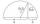Calculate the geometric mean of numbers a=15.2 and b=25.6. Determine the mean by construction where a and b are the length of the lines.
• Two geometric progressions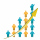Insert several numbers between numbers 6 and 384 so that they form with the given GP numbers and that the following applies: a) the sum of all numbers is 510 And for another GP to apply: b) the sum of entered numbers is -132 (These are two different geome
• Three members GPThe sum of three numbers in GP (geometric progression) is 21 and the sum of their squares is 189. Find the numbers.
• Six speeds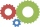A drilling machine is to have 6 speeds ranging from 50 to 750 revolutions per minute. If the speed forms a geometric progression, determine their values.
• Geometric sequenceIn the geometric sequence is a4 = 20 a9 = -160. Calculate the first member a1 and quotient q.
• If the 3If the 6th term of a GP is 4 and the 10th is 4/81, find common ratio r.
• Sum of seriesDetermine the 6-th member and the sum of a geometric series: 5-4/1+16/5-64/25+256/125-1024/625+....
• What areWhat are the four terms between 4 and 19?
• Negative difference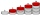Find four arithmetic progression members between 7 and -6.
• Geometric sequence 3In geometric sequence is a8 = 312500; a11= 39062500; sn=1953124. Calculate the first item a1, quotient q, and n - number of members by their sum s_n.
• Sum 1-6Find the sum of the geometric progression 3, 15, 75,… to six terms.
• Geometric progressionIn geometric progression, a1 = 7, q = 5. Find the condition for n to sum first n members is sn≤217.
• Geometric progressiobIf the sum of four consective terms of geometric progression is 80 and arithmetic mean of second and fourth term is 30 then find terms?